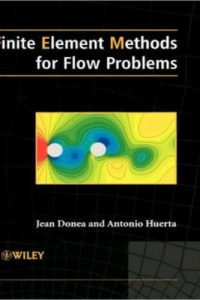اسم المؤلف
Jean Donea and Antonio Huerta
التاريخ
9 فبراير 2019
المشاهدات
التقييمLoading...

Finite Element Methods for Flow Problems
Jean Donea and Antonio Huerta
Preface xi
1 Introduction and preliminaries 1
1.1 Finite elements in fluid dynamics 1
1.2 Subjects covered 2
1.3 Kinematical descriptions of the flow field 4
1.3.1 Lagrangian and Eulerian descriptions 5
1.3.2 ALE description of motion 8
1.3.3 Thefundamental ALE equation 11
1.3.4 Time derivative of integrals over moving
volumes 12
1.4 The basic conservation equations 13
1.4.1 Mass equation 13
1.4.2 Momentum equation 13
1.4.3 Internal energy equation 15
1.4.4 Total energy equation 17
1.4.5 ALE form of the conservation equations 18
1.4.6 Closure of the initial boundary value problem 19
1.5 Basic ingredients of the finite element method 19
1.5.1 Mathematical preliminaries 19
ContentsVi CONTENTS
1.5.2 Trial solutions and weighting functions 21
1.5.3 Compact integralforms 23
1.5.4 Strong and weak forms of a boundary value
problem 23
1.5.5 Finite element spatial discretization 27
2.1 Problem statement 33
2.1.1 Strongform 33
2.1.2 Weak form 35
2.2 Galerkin approximation 36
2.2.1 Piecewise linear approximation in 1D 37
2.2.2 Analysis of the discrete equation 40
2.2.3 Piecewise quadratic approximation in ID 45
2.2.4 Analysis of the discrete equations 47
2.3 Early Petrov—Galerkin methods 50
2.3.1 Upwind approximation of the convective term 50
2.3.2 First finite elements of upwind type 51
2.3.3 The concept of balancing diffusion 53
2.4 Stabilization techniques 59
2.4.1 The SUPG method 60
2.4.2 The Galerkin/Least-squares method 63
2.4.3 The stabilization parameter 64
2.5 Other stabilization techniques and new trends 65
2.5.1 Finite increment calculus 66
2.5.2 Bubble Junctions and wavelet approximations 67
2.5.3 The variational multiscale method 68
2.5.4 Complements 70
2.6 Applications and solved exercises 70
2.6.1 Construction of a bubblefunction method 70
2.6.2 One-dimensional transport 72
2.6.3 Convection—diffusion across a source term 74
2.6.4 Convection—diffusion skew to the mesh 75
2.6.5 Convection—diffusion-reaction in 2D 76
2.6.6 The Hemker problem 78
3.1 Introduction 79
3.2 Problem statement 81CONTENTS vii
3.3 The method of characteristics 32
3.3.1 The concept of characteristic lines 82
3.3.2 Properties of the linear convection equation 84
3.3.3 Methods based on the characteristics 87
3.4 Classical time and space discretization techniques 91
3.4.1 Time discretization 92
3.4.2 Galerkin spatial discretization 94
3.5 Stability and accuracy analysis 98
3.5.1 Analysis of stability by Fourier techniques 99
3.5.2 Analysis of classical time-stepping schemes 101
3.5.3 The modified equation method 105
3.6 Taylor—Galerkin Methods 107
3.6.1 The needfor higher-order time schemes 107
3.6.2 Third-order explicit Taylor—Galerkin method 108
3.6.3 Fourth-order explicit leap-frog method 113
3.6.4 Two-step explicit Taylor—Galerkin methods 114
3.7 An introduction to monotonicity-preserving schemes 117
3.8 Least-squares-based spatial discretization 120
3.8.1 Least-squares approach for the 6family of
methods 121
3.8.2 Taylor least-squares method 122
3.9 The discontinuous Galerkin method 124
3.10 Space—time formulations 126
3.10.1 Time-discontinuous Galerkinformulation 126
3.10.2 Time-discontinuous least-squaresformulation 128
3.10.3 Space-timeGalerkin/Least-squaresformulation 128
3.11 Applications and solved exercises 129
3.11.1 Propagation of a cosine profile 129
3.11.2 Travelling wave package 133
3.11.3 The rotating cone problem 135
3.11.4 Propagation of a steepfront 137
Compressible Flow Problems 147
4.1 Introduction 147
4.2 Nonlinear hyperbolic equations 149
4.2.1 Scalar equations 149
4.2.2 Weak solutions and entropy condition 152
4.2.3 Time and space discretization 156
4.3 The Euler equations 159Viii CONTENTS
4.3.1 Strongform of the conservation equations 159
4.3.2 The quasi-linear form of the Euler equations 161
4.3.3 Basic properties of the Euler equations 163
4.3.4 Boundary conditions 165
4.4 Spatial discretization techniques 166
4.4.1 Galerkinformulation 167
4.4.2 Upwind-type discretizations 168
4.5 Numerical treatment of shocks 176
4.5.1 Introduction 176
4.5.2 Early artificial diffusion methods 178
4.5.3 High-resolution methods 180
4.6 Nearly incompressible flows 186
4.7 Fluid-structure interaction 187
4.7.1 Acoustic approximation 189
4.7.2 Nonlinear transient dynamic problems 191
4.7.3 Illustrative examples 196
4.8 Solved exercises 199
4.8.1 One-step Taylor—Galerkin solution of Burgers’
equation 199
4.8.2 The shock tube problem 202
5.1 Introduction 209
5.2 Problem statement 210
5.3 Time discretization procedures 211
5.3.1 Classical methods 211
5.3.2 Fractional-step methods 213
5.3.3 High-order time-stepping schemes 215
5.4 Spatial discretization procedures 222
5.4.1 Galerkin formulation of the semi-discrete
scheme 222
5.4.2 Galerkinformulation of 0family methods 224
5.4.3 Galerkinformulation of explicit Pade schemes 228
5.4.4 Galerkin formulation of implicit multistage
schemes 229
5.4.5 Stabilization of the semi-discrete scheme 231
5.4.6 Stabilization of multistage schemes 233
5.5 Stabilized space-time formulations 241
5.6 Solved exercises 243CONTENTS IX
5.6.1 Convection-diffusion of a Gaussian hill 243
5.6.2 Transient rotating pulse 246
5.6.4 Nonlinear propagation of a step 250
5.6.5 Burgers’ equation in 1D 251
5.6.6 Two-dimensional Burgers’ equation 252
Appendix Least-squares in transient/relaxation problems 254
Viscous incompressible flows 265
6.1 Introduction 265
6.2 Basic concepts 267
6.2.1 Strain rate and spin tensors 267
6.2.2 The stress tensor in a Newtonian fluid 268
6.2.3 The Navier—Stokes equations 269
6.3 Main issues in incompressible flow problems 272
6.4 Trial solutions and weighting functions 273
6.5 Stationary Stokes problem 275
6.5.1 Formulation in terms of Cauchy stress 275
6.5.2 Formulation in terms of velocity and pressure 278
6.5.3 Galerkinformulation 279
6.5.4 Matrix problem 281
6.5.5 Solvability condition and solution procedure 283
6.5.6 The LBB compatibility condition 284
6.5.7 Some popular velocity—pressure couples 285
6.5.8 Stabilization of the Stokes problem 287
6.5.9 Penalty method 288
6.6.1 Weak form and Galerkinformulation 293
6.6.2 Matrix problem 293
6.7.1 Weak formulation and spatial discretization 295
6.7.2 Stabilized finite elementformulation 296
6.7.3 Time discretization byfractional-step methods 297
6.8 Applications and solved exercices 306
6.8.1 Stokes flow with analytical solution 306
6.8.2 Cavity flow problem 307
6.8.3 Planejet simulation 313
6.8.4 Natural convection in a square cavity 317
كلمة سر فك الضغط : books-world.net